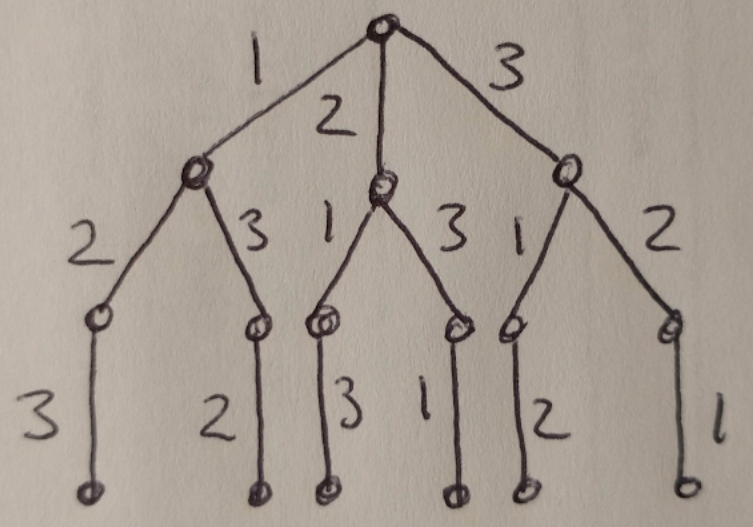# Backtracking Algorithm

I have been working through Leetcode’s Algorithm I study plan and recently came across the backtracking set of problems (days 10 and 11) . I wanted to give a brief explanation of what backtracking is, and how it uses other CS tools like tree graphs and depth-first search.

The basic idea behind backtracking is that we want to find all possible solutions to a problem using brute force, and then discard all the solutions that don’t meet certain criteria established by the problem definition . As you might imagine, this is not a very efficient algorithm so do not use it if you need to meet time or memory constraints . That being said, let’s take a closer look at how the algorithm works on a toy example.

Let’s find all the possible permutations of the numbers {1, 2, 3} where 1 and 3 are not adjacent. First, we need to find all of the possible solutions to this problem (we’ll eliminate the ones that don’t meet the adjacency constraint in a second step). We can do this using a tree to represent the state space, i.e. all the possible solutions . This tree is shown in Figure 1.Figure 1

Every branch of this tree represents one of the possible solutions to this problem (i.e. a unique state). We can use depth-first search (DFS) to list out all the possible solutions: [1, 2, 3], [1, 3, 2], [2, 1, 3], [2, 3, 1], [3, 1, 2], [3, 2, 1]. (Notice that there are 6 solutions and 6 branches in the tree.) But what do we do about the fact that some of these solutions place 1 and 3 next to each other? We could add a check in our DFS algorithm such that if the constraint is violated (i.e. we find [1, 3 …]), then we stop searching along this branch and return to the root node to try the next branch instead . This method would leave us with the 2 viable solutions: [1, 2, 3] and [3, 2, 1].

We can use backtracking to solve several kinds of problems, including decision problems (where we are trying to answer a yes/no question ), optimization problems (where we are trying to find the best answer to a problem with multiple solutions ) or enumeration problems like the one above where we are finding a set of permutations/solutions to a problem .

 “Algorithm I Study Plan.” Leetcode. https://leetcode.com/study-plan/algorithm/ Visited 4 Jan 2022.

 Datta, S. “Backtracking Algorithms.” Baeldung CS. 26 Nov 2020. https://www.baeldung.com/cs/backtracking-algorithms Visited 4 Jan 2022.

 “Decision problem.” Wikipedia. https://en.wikipedia.org/wiki/Decision_problem Visited 4 Jan 2022.